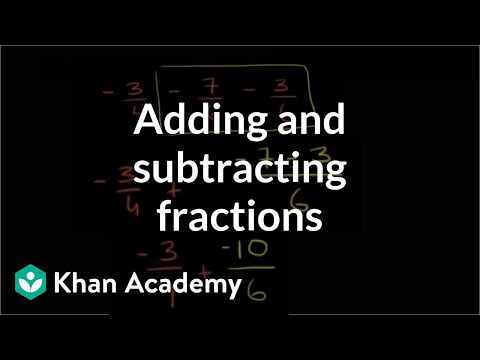Video

# Adding & subtracting negative numbers (Full video)

Description: Learn how to add and subtract negative numbers. The problems solved in this video are 2 - 3 = -1 and -2 - 3 = -5 and -2 + 3 = 1 and 2 - (-3) = 5 and -2 - (-3). Created by Sal Khan. Let's have some practice adding and subtracting negative numbers. And I'm going to do it with a number line. So this is 2 right over here, and then we're going to subtract 3 from that 2.

### Other videos you might be interested in### Absolute value as distance between numbers (Full video)